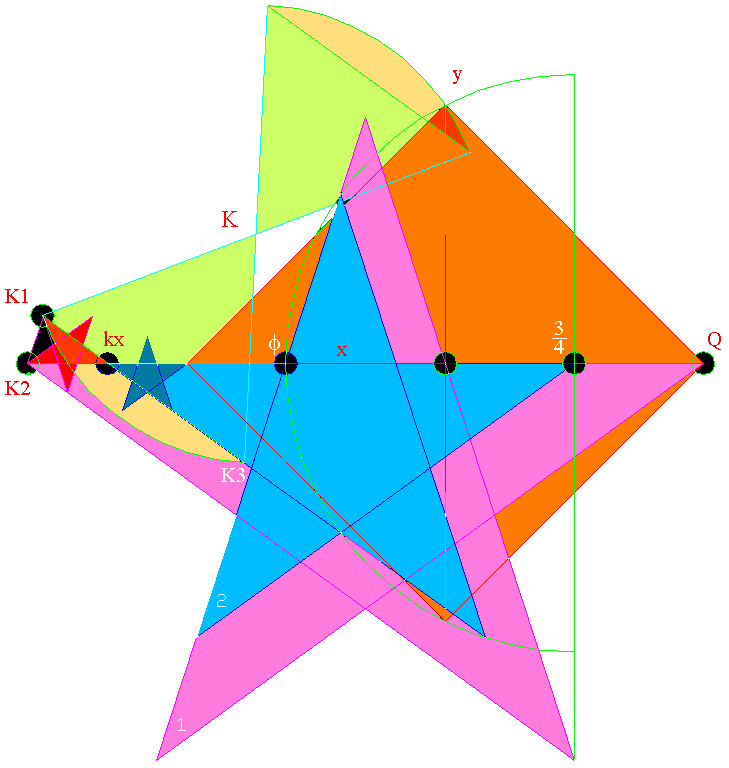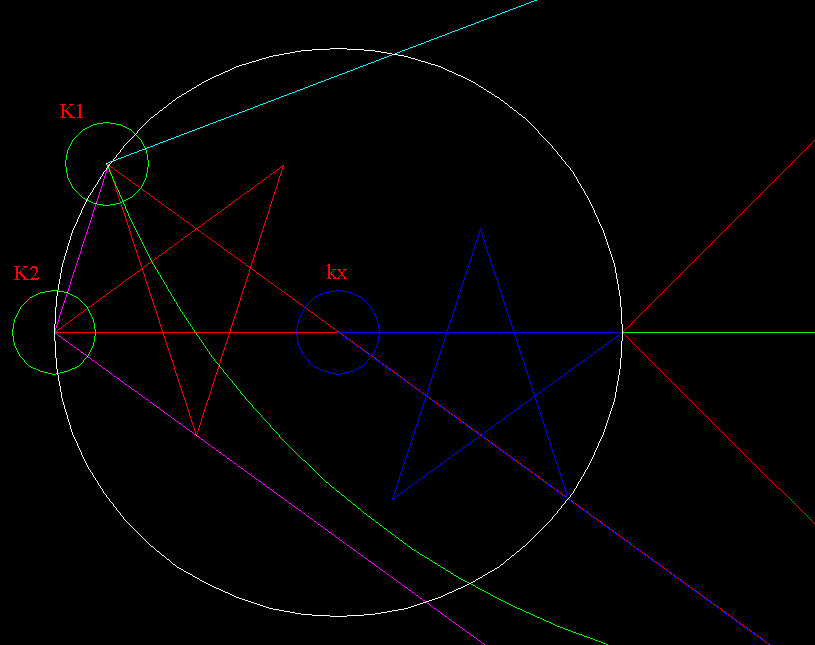Top       Nasca Monkey      Gallery
Frame 1   * 2     * 3     Articles    Continue

# kx-stars - a Feature of the Seal of Atlantis

The line k  passing through K1 and K3 is a part of the hourglass form
within the Cone's Key (Top) circle. Remarkably, it  holds a 36 degree angle
with the X-axis. Usually, such an angle implies a unique five-pointed star,
which fits either the art, or the geometry given by the same art, if not both.
Is this position set-up for a unique meaningful pentagram, as well?
*
It is the Square's x-axis, which forms a 36-degree angle with the line k.
Also, it is visually just as far from the point of kx to the point K1, as it is
to the Square's nearest corner. So we pick this nearest point of the Square
to size our first experimental star.  The fact is interesting and eye pleasing.
Next, we wouldn't want to miss the order shown in the diagram below.When one half of the Square's diagonal is counted as 1, when extended
to the Phi-point, this distance becomes 1.6180339887.. i.e., Phi. .
A circle of the radius 0.6180339887.. will map exactly ten times
onto the Square's circumcircle, as this is the method of constructing
both the five and ten-pointed stars.

The Phi-point also performs Golden Section on the length kx -  3/4 point
of the Square's diagonal.

Similarly, a star drawn from the symmetrical point K2 to the far corner
of the Square proves to be part of the same system. Both the Square's
center and the Phi-point serve to divide the distance from K2 to Q in the
Golden Ratio.. We are looking at a diagram of a classic geometrical position.The idea of the Cone's Key-circle is a nice addition to our collection
of variations on the Golden Section. The diagram looks precise even
when blown-up as above, though in reality it is off by a couple of
thousandths of our unit. I suppose, what matters is we that we were
shown a simulation of another perfect design having to do with the
Square's PHI-constructions.

continue       Top       Nasca Monkey     Namon's Gallery      Frame 1   * 2     * 3     Articles